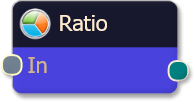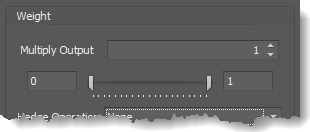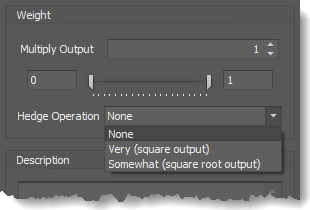#### Risk Disclosure

Futures, foreign currency and options trading contains substantial risk and is not for every investor. An investor could potentially lose all or more than the initial investment. Risk capital is money that can be lost without jeopardizing ones financial security or lifestyle. Only risk capital should be used for trading and only those with sufficient risk capital should consider trading. Past performance is not necessarily indicative of future results. View full risk disclosure.

# Ratio NodeThe Ratio logic node takes each of the inputs and calculates a weighted average of the values to produce an output.  This is useful for implementing a system where the optimal combination of influence for each criteria is unknown.  You can use the Ratio logic node in conjunction with the output multiplier (found on the weight slider) to fine tune the relative effect each criteria will have on the final output.

Ratio logic nodes take full advantage of the fuzzy output generated by solvers.  In a fuzzy logic system where values are presented in a range between 0 and 1 (0% to 100%), the Ratio logic node allows you to combine the influence of your criteria in an analog fashion.  This is best used for those systems where you have outlined a number of positive factors contributing to a trade signal, but there are instances where a deficiency in one criteria can be compensated by another.  The Ratio logic node emulates the discretionary trader who examines a variety of positive (or negative) factors weighing the contribution of each of them before executing a trade.

### Example

Suppose you had several criteria but needed the flexibility to change how much each individual criteria contributes to the final output.

• Criteria #1
• Criteria #2
• Criteria #3
• Criteria #4

After adding the solvers to the Logic Board work area, add a Ratio node. Then connect the outputs of each solver to the input points on the Ratio node.  Finally, connect the Ratio node to the (green) Result node.  You should notice immediately that BloodHound’s output appears to be a blend or average value of all the criteria combined (connected solvers).

At this point you can manipulate the Options tab » Weighting to increase or reduce the influence of each solver separately.  Suppose that you would like to increase the influence of criteria #1 so that its favorable value was twice as important as the other criteria.  To do this, you would click on the solver for criteria #1 to select it.  In the Property Inspector (right hand panel), select the Options tab, were you will see the weight slider.  Set the Multiplier Output to 2.0.  Criteria #1 is now weighted as twice as significant as the other criteria, giving it more influence over the Ratio node’s final output.

As you change the influence, you will notice that BloodHound immediately updates the chart with the new result.  This allows you to make tweaks and visually inspect the results until you are satisfied.  See the section on weight slider control to learn how to do this.

After changing the weighting for your solvers, suppose that you now have the following Multiplier Output (weights) values for each input to the Ratio node:

Criteria Weight
Input #1 1.5
Input #2 2
Input #3 0.5
Input #4 1

The resulting calculation on each bar would be derived from the following formula (which is simply a weighted average calculation, using the Output Multiplier as the weighting):# BloodHound   v2

This section is specific to BloodHound v2 Property Inspector's elements.  The node functions exactly the same in BloodHound v1 and v2.

## Properties tab

This tab contains the essential settings for all nodes.

### Modifiers

Please note:   The buttons only modify the individual instance of the selected node(s) on a Logic Board.   Thus, the buttons are only visible when a node is selected on the Logic Board.  The buttons are not available when a solver is selected in the Solvers panel, because the original solver's output can not be globally modified.
This behavior is consistent with adding a function node afterwards, so that the original solver's output remains unmodified elsewhere in the system.  It is similar to having an SMA(50) on several charts.  Changing the plot color on one chart does not modify the plot color on the other charts.

## Options tab

This tab contains extra secondary settings common to all nodes.

### Weight

This section is used to modify the node's final output value.  It is only useful for designing fuzzy logic systems, or a fuzzy logic section of a system.
Please note:   The Weight controls only modify the individual instance of the selected node(s) on a Logic Board.   Thus, the Weight controls are only visible when a node is selected on the Logic Board.  The controls are not available when a solver is selected in the Solvers panel, because the original solver's output can not be globally modified.
This behavior is different than BloodHound 1.0.  In BH 1.0, the Weight controls modified the nodes output globally (every instance).  This change in BH 2.0 adds more system design granularity.Multiply Output:   This takes the internal values (the Long and Short values determined via the Properties tab » Output Rules section) and multiplies them by this value.  Note: the final output will not exceed a value of 1, as described in the Slider control below.

Slider control:  The slider constrains the final output to a value of 0 to 1.  The left side of the slider sets a minimum value that is output regardless if the solver condition is true or not.  The right side sets a maximum value that is output.  The output is capped.
e.g.  Three indicator conditions are being checked, and thus three solvers are created.  Only two out of the three indicator conditions are needed.  An Additive logic node is used to add the solver's outputs together.  Just two out of the three solvers need to add up to a value of 1.  Therefore, the right slider (max output value) for all three solvers is set to 0.5.  When two indicator/solver conditions are found, thus the outputs = 0.5, then the calculation, in the Additive node, is 0.5 + 0.5 + 0 = 1.  A value of 1 means the two out of three condition is true.Hedge Operation: This applies a mathematical formula to the internal value.

None:  No modification is applied.
Very (square output):  A squaring formula is applied.  Output  =  value .
Somewhat (square root output):  A square root formula is applied.  Output  =  √value .

### Description

This text area provides a place to write a full description of what the node is doing, used for, or what ever you want.
Note:   The Description is global to all instances of the node.  It is not applied individually to each instance as the Weight controls are.

## Help tab

This tab displays the documentation page (from this web site) of the selected node.
Please note:  NinjaTrader v8.0.26.0 or newer is required for the built in web viewer to work, and thus the documentation to be displayed.

## Example Video

This video is a snippet from the workshop on January 6, 2017.  Find all workshop recordings here.

#### Risk Disclosure

Futures, foreign currency and options trading contains substantial risk and is not for every investor. An investor could potentially lose all or more than the initial investment. Risk capital is money that can be lost without jeopardizing ones financial security or lifestyle. Only risk capital should be used for trading and only those with sufficient risk capital should consider trading. Past performance is not necessarily indicative of future results. View full risk disclosure.

# Ratio NodeThe Ratio logic node takes each of the inputs and calculates a weighted average of the values to produce an output.  This is useful for implementing a system where the optimal combination of influence for each criteria is unknown.  You can use the Ratio logic node in conjunction with the output multiplier (found on the weight slider) to fine tune the relative effect each criteria will have on the final output.

Ratio logic nodes take full advantage of the fuzzy output generated by solvers.  In a fuzzy logic system where values are presented in a range between 0 and 1 (0% to 100%), the Ratio logic node allows you to combine the influence of your criteria in an analog fashion.  This is best used for those systems where you have outlined a number of positive factors contributing to a trade signal, but there are instances where a deficiency in one criteria can be compensated by another.  The Ratio logic node emulates the discretionary trader who examines a variety of positive (or negative) factors weighing the contribution of each of them before executing a trade.

### Example

Suppose you had several criteria but needed the flexibility to change how much each individual criteria contributes to the final output.

• Criteria #1
• Criteria #2
• Criteria #3
• Criteria #4

After adding the solvers to the Logic Board work area, add a Ratio node. Then connect the outputs of each solver to the input points on the Ratio node.  Finally, connect the Ratio node to the (green) Result node.  You should notice immediately that BloodHound’s output appears to be a blend or average value of all the criteria combined (connected solvers).

At this point you can manipulate the Options tab » Weighting to increase or reduce the influence of each solver separately.  Suppose that you would like to increase the influence of criteria #1 so that its favorable value was twice as important as the other criteria.  To do this, you would click on the solver for criteria #1 to select it.  In the Property Inspector (right hand panel), select the Options tab, were you will see the weight slider.  Set the Multiplier Output to 2.0.  Criteria #1 is now weighted as twice as significant as the other criteria, giving it more influence over the Ratio node’s final output.

As you change the influence, you will notice that BloodHound immediately updates the chart with the new result.  This allows you to make tweaks and visually inspect the results until you are satisfied.  See the section on weight slider control to learn how to do this.

After changing the weighting for your solvers, suppose that you now have the following Multiplier Output (weights) values for each input to the Ratio node:

Criteria Weight
Input #1 1.5
Input #2 2
Input #3 0.5
Input #4 1

The resulting calculation on each bar would be derived from the following formula (which is simply a weighted average calculation, using the Output Multiplier as the weighting):The Ratio node in BloodHound v1 and v2 function exactly the same. The only difference is how the node is visualized in the Property Inspector.  See the BloodHound v2 section above for a description and example.

## Example Video

This video is a snippet from the workshop on January 6, 2017.  Find all workshop recordings here.

#### Risk Disclosure

Futures, foreign currency and options trading contains substantial risk and is not for every investor. An investor could potentially lose all or more than the initial investment. Risk capital is money that can be lost without jeopardizing ones financial security or lifestyle. Only risk capital should be used for trading and only those with sufficient risk capital should consider trading. Past performance is not necessarily indicative of future results. View full risk disclosure.

# Ratio NodeThe Ratio logic node takes each of the inputs and calculates a weighted average of the values to produce an output.  This is useful for implementing a system where the optimal combination of influence for each criteria is unknown.  You can use the Ratio logic node in conjunction with the output multiplier (found on the weight slider) to fine tune the relative effect each criteria will have on the final output.

Ratio logic nodes take full advantage of the fuzzy output generated by solvers.  In a fuzzy logic system where values are presented in a range between 0 and 1 (0% to 100%), the Ratio logic node allows you to combine the influence of your criteria in an analog fashion.  This is best used for those systems where you have outlined a number of positive factors contributing to a trade signal, but there are instances where a deficiency in one criteria can be compensated by another.  The Ratio logic node emulates the discretionary trader who examines a variety of positive (or negative) factors weighing the contribution of each of them before executing a trade.

### Example

Suppose you had several criteria but needed the flexibility to change how much each individual criteria contributes to the final output.

• Criteria #1
• Criteria #2
• Criteria #3
• Criteria #4

After adding the solvers to the Logic Board work area, add a Ratio node. Then connect the outputs of each solver node to the input points on the Ratio node, and finally connect the Ratio node itself to the Result node. You should notice immediately that BloodHound’s output appears to be a blend or average value of all the criteria combined.

At this point you can manipulate the output multiplier for each solver node to increase or reduce the influence of each solver separately. Suppose that you would like to increase the influence of criteria 1 so that it’s favorable value was twice as meaningful as the other criteria. To do this, you would click on the Ratio node to select it. On the right hand pane, you will see a list of weight sliders, each pertaining to the solver(along with the weight slider for the Ratio node itself at the top). Find the weight slider for criteria 1, and set the output multiplier to 2.0. Criteria 1 is now weighted as twice as significant as the other criteria, giving it more influence over the Ratio node’s final output.

As you change the influence in real-time mode, you will notice that BloodHound immediately updates the chart with the new weightings. This allows you to make tweaks  and visually inspect the results until you are satisfied. Another tip to observe is that you can assign a plot color to each of the solvers to inspect their raw values while you are manipulating their weights. See the section on weight slider control to learn how to do this.

After changing the weighting for your solvers, suppose that you now have the following output multiplier(weights) values for each input to the Ratio node:

Criteria Weight
Input #1 1.5
Input #2 2
Input #3 0.5
Input #4 1

The resulting calculation on each bar would be derived from the following formula (which is simply a weighted average calculation, using the Output Multiplier as the weighting):## Example Video

This video is a snippet from the workshop on January 6, 2017. Find all workshop recordings here.